Courses

# Test: Thermal Expansion

## 10 Questions MCQ Test Physics Class 11 | Test: Thermal Expansion

Description
This mock test of Test: Thermal Expansion for JEE helps you for every JEE entrance exam. This contains 10 Multiple Choice Questions for JEE Test: Thermal Expansion (mcq) to study with solutions a complete question bank. The solved questions answers in this Test: Thermal Expansion quiz give you a good mix of easy questions and tough questions. JEE students definitely take this Test: Thermal Expansion exercise for a better result in the exam. You can find other Test: Thermal Expansion extra questions, long questions & short questions for JEE on EduRev as well by searching above.
QUESTION: 1

### Two identical rectangular strips, one of copper and the other of steel, are riveted as shown to form a bi-metal strip. On heating, the bi-metal strip will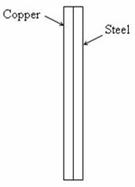Solution:

On heating, the copper strip will suffer greater elongation and hence the bimetal strip will bend with the steel strip on the concave side.

[Bimetal strips are widely used in thermal switching applications such as automatic electric iron].

QUESTION: 2

### Four cylindrical rods of different radii and lengths are used to connect two heat reservoirs at fixed temperatures t1 and t2 respectively. From the following pick out the rod which will conduct the maximum quantity of heat:

Solution:

The rate of heat transfer is directly proportional to the bisectional surface area of the solid and inversely proportional to its parallel length.
That is heat conduction rate say H ∝ r2
∝ 1/L
I.e ∝ r2/L
Hence the conduction would be
maximum in case in which  r2/L ratio is largest.

QUESTION: 3

### The temperature for which the reading on Celsius and Fahrenheit scales are identical is

Solution:

The Celsius and Fahrenheit are two important temperature scales. The Fahrenheit scale is used primarily in the United States, while Celsius is used throughout the world. The two scales have different zero points and the Celsius degree is bigger than the Fahrenheit one. There is one point on the Fahrenheit and Celsius scales where the temperatures in degrees are equal. This is -40 degree C and -40 degree F.

QUESTION: 4

A steel tape is calibrated at 20° C. A piece of wood is being measured by steel tape at 10°C and reading is 30 cm on the tape. The real length of the wood is:

Solution:

When heated the length between adjacent markings increases. Hence the actual length will be less than
30 cm.

QUESTION: 5

Which phase of matter has maximum value of temperature coefficient of cubical expansion?

Solution:

Gas expands more than solid and liquid because gas particles are far apart hence freely move.

QUESTION: 6

The coefficient of liner expansion of a cubical crystal along three mutually perpendicular direction is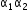and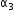. What is the coefficient of cubical expansion of crystal?

Solution:

Cubical expansion means there is expansion is length (a1), breadth (a2) and height (a3). Therefore the net coefficient of cubical expansion is α1 + α2 + α3.

QUESTION: 7

Relationship for converting the Fahrenheit and Celsius scales is

Solution: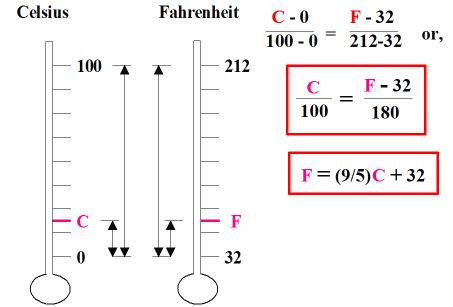QUESTION: 8

When water is heated from 0° C to 20° C its volume:

Solution:

When water is heated from 0°C, its volume decreases because its density increases and you can see this effect upto 4°C. Since the density of ice is maximum at 4°C, afterwards as the density decreases the volume increases. The main reason for this is hydrogen bond in ice gets cleaved due to the melting of ice.

QUESTION: 9

The relation between coefficient of volume expansion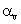and coefficient of linear expansion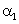is

Solution:

This is simple because the coefficients of linear expansion αL, areal expansion αA, and volume expansion αv, are in the ratio of 1: 2: 3 i.e.:
αL: αA: αv :: 1: 2: 3
Hence, αv = 3αL

QUESTION: 10

Water contract on heating between the temperatures

Solution:

When water is heated from 0oC, its volume decreases because its density increases and you can see this effect upto 4oC. Since the density of ice is maximum at 4oC, afterwards as the density decreases the volume increases. The main reason for this is hydrogen bond in ice gets cleaved due to the melting of ice.